# Theory & Procedure, Equivalent Resistance of Resistors (Series) Notes | Study Science Class 10 - Class 10

## Class 10: Theory & Procedure, Equivalent Resistance of Resistors (Series) Notes | Study Science Class 10 - Class 10

The document Theory & Procedure, Equivalent Resistance of Resistors (Series) Notes | Study Science Class 10 - Class 10 is a part of the Class 10 Course Science Class 10.
All you need of Class 10 at this link: Class 10

Our Objective

How to determine the equivalent resistance of two resistors when connected in series.

The Theory

Before we start learning about series circuits, we need to know what electrical circuits are and what are different types of circuits!

Electrical Circuit

An electric circuit is simply a closed loop through which charges can continuously move. An electric circuit basically contains a source of electricity, a load resistance, a switch or a key for turning the circuit on or off at one's convenience.

The diagrammatic representation of an electric circuit is called the circuit diagram.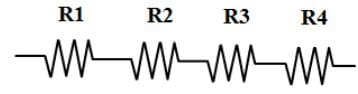Types of circuits

When two or more resistors are connected such a way that one end of one resistor is connected to the starting end of the other, then the circuit is called a Series Circuit

Similarly, if the starting ends of two resistors are joined to a point and the terminal ends of the two are combined and given connection to a source of electricity,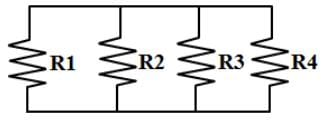those circuits are called Parallel Circuit.

Since the current has only one path to take, the current through a series circuit is the same through each resistor.

Before calculating the equivalent resistance, let me ask you, "What is resistance?"

Voltage can be thought of as the pressure pushing charges along a conductor.

Electrical resistance shows how much energy you need when you move the charges/current through your devices. If you need lots of energy, then the resistance required is also high.

In many materials, the voltage and resistance are connected by Ohm's Law: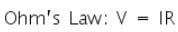That is,  the property of a conductor to oppose the flow of charges through it is called its resistance.
It is measured as resistance,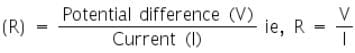All metals are good conductors, so all metal wires have a relatively low resistance.

The longer a piece of wire the higher is its resistance. Double the length means double the resistance. R ∝ I ie.,

The resistance of a piece of wire also depends on its thickness/cross-sectional area A of the conductor. Double the area means half the resistance.  A thin wire has a higher resistance than the same thick piece.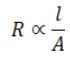ie.,

Finally, resistance of a piece of wire depends on its temperature also. The higher the temperature the higher is the resistance.

Calculating the effective resistance/total resistance of series circuits:

The total resistance/effective resistance of the circuit is found by simply adding up the resistance values of the   individual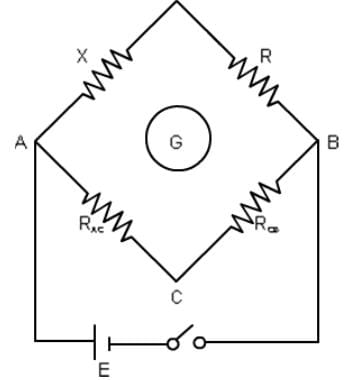resistors. Adding resistors in series always increases the effective resistance.
ie.,  If  Rs  be the resistance of the series combination then,
R5 = R1 + R2 + R3 + ......

Here, we used Meter Bridge to calculate the effective resistance accurately.

The Meter Bridge is a device for measurement of resistance using the principle of Wheatstone Network.

Let’s do an experiment now ……

Materials Required: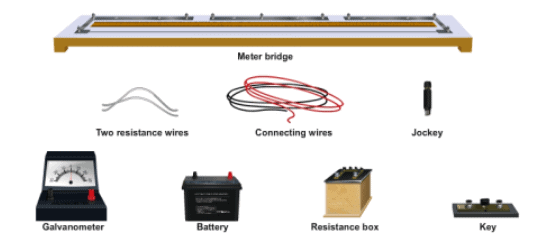Procedure:

As performed in the real lab:

1. Make a circuit as shown in the figure.
2. Ensure that all the connections are tight.
3. Check whether the connections are correct by removing some resistance from the resistance box and close the plug key ‘K’.
4. Press the jockey at the left extreme of the metre bridge. Mark the direction of the deflection on the galvanometer. Again press the jockey at right extreme of the metre bridge and mark the direction of the deflection again. If the directions of the deflections are in opposite direction, then the connections are correct, otherwise check the connections.
5. The balancing point is obtained by sliding the jockey along the wire and when there is no deflection in the galvanometer.
6. Take at least three sets of observations by moving the jockey from both the end of the bridge for each wire and also when it is serially connected.
8. Find its resistance of this combination as mentioned in the below observation table.

As performed in the simulator: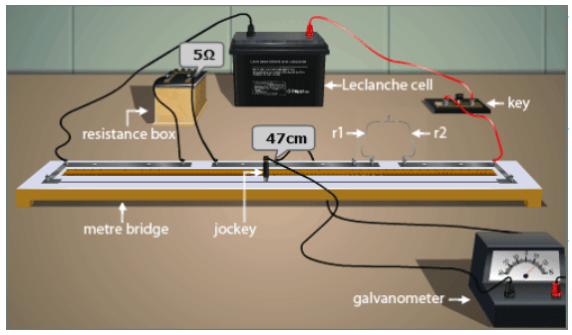1. Your simulator will consist of a metre bridge kept on a table, battery, resistance box and wires on the side bar menu.
2. You can calculate the resistance of a single wire or serially connected wire by selecting from the drop down menu, “Arrangement of Resistors”.
3. If you selected, “Single”, then drag the battery and the resistance box shown on the side bar menu near to the metre bridge using your mouse.
4. Drag one of the wires to the right gap of the metre bridge.
5. Now the button, “Start experiment” will be enabled.
6. Now you can select your desired resistance from the resistance box just by clicking on the box and then choosing the resistance from the pop-window, “Select Resistance”. Now close the pop-window.
7. Click on the enabled button and "Insert Key”.
8. Now you can move the jockey from one left end to right either by moving the jockey with your mouse or by moving the slider, “Jockey Position”.
9. Simultaneously check the readings of the galvanometer, once the needle reaches the zero reading, stop moving the jockey and note down the length of the wire from the balanced position on the left side, let say “AB” which is l cm.
10. Repeat the same by moving the jockey from the right end to the left and note down the length of the wire from the balanced position on the right side, let take it as “BC” which is 100-l cm.
11. Repeat the same procedure with second wire and note down the lengths.
12. For each wire take three readings and calculate its mean readings/resistance.
13. Repeat the same procedures for the series connection.

Calculations:

1. Calculation for ronly, r2 only, r1 and r2 in series
2. Calculation for verification of laws in series

Experimental value of Rs               = ........... ohm
Theoretical value of Rs       =   r1 + r2 = ........... ohm
Difference (if any)                        = ........... ohm

Result:

Within the limits of experimental error, experimental and theoretical values of Rs are same. Hence, law of resistance in series is verified.

The document Theory & Procedure, Equivalent Resistance of Resistors (Series) Notes | Study Science Class 10 - Class 10 is a part of the Class 10 Course Science Class 10.
All you need of Class 10 at this link: Class 10Use Code STAYHOME200 and get INR 200 additional OFF

## Science Class 10

85 videos|341 docs|162 tests

Track your progress, build streaks, highlight & save important lessons and more!

,

,

,

,

,

,

,

,

,

,

,

,

,

,

,

,

,

,

,

,

,

,

,

,

;